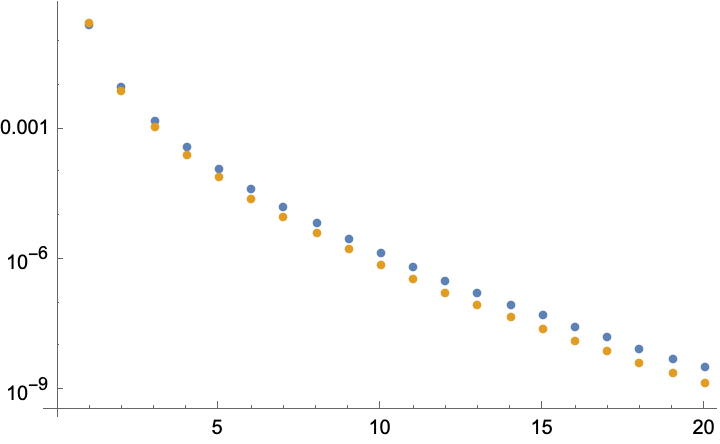# Asymptotics of the integral of an oscillating function

I would like to know the asymptotics of the following sequences of integrals: $$I_n = \displaystyle { \int _0 ^{+ \infty} \dfrac{t^n}{(t + i)^{n + 1}} \cdot e^{-t} \ dt }$$

Using a deformation of path with Cauchy theorem, I've shown that $$I_n = \displaystyle { \int _{i \mathbb{R}^+} \dfrac{t^n}{(t + i)^{n + 1}} \cdot e^{-t} \ dt = \int _0 ^{+ \infty} \dfrac{t^n}{(t + 1)^{n + 1}} \cdot e^{-it} \ dt }$$

I therefore have tried using Laplace method, but I have been unable to conclude anything...

Moreover, I didn't manage to use computer software to have some reliable numeric values, because of the oscilating function $$t \mapsto e^{-it}$$. So, I haven't got any conjecture...

The saddle point $$t^\ast$$ is obtained by solving $$f'(t)=0$$ for $$f(t)=-t+n\ln t-(n+1)\ln(t+i)$$, for large $$n$$ we find $$f(t^\ast)=-2\sqrt{in}$$, so we arrive at the approximation for the integral $$I_n\approx\exp(-2\sqrt{in})$$.
The plot compares exact (gold) and approximate (blue) absolute values for $$n$$ up to 200 (multiply horizontal axis labels by 10).• Nice cart, to confirm theory! I find two possibilities to the saddle point $t^\star$: $\dfrac{-1 - i \pm \sqrt{2i (2n + 1)}}{2}$. Which one should we consider? – MathTolliob Jun 15 at 18:35
• Do you have a link on with theory and example on Laplace method? I thought it applies on integral $\displaystyle { \int _a ^b g(t) e^{-\lambda f(t)} \ dt }$, with a function $f$ non-depending on the variable $\lambda$? – MathTolliob Jun 15 at 18:35
• Using the saddle node $\dfrac{-1 -i + \sqrt{2i(2n + 1)}}{2}$, I conjecture that $I_n \underset{n \longrightarrow + \infty}{\sim} \dfrac{\sqrt{\pi}}{n^{\frac{1}{4}}} e^{i \big ( \frac{1}{2} - \frac{\pi}{8} \big )} e^{-2 \sqrt{i n}}$. This has been confirmed by numerical result for $n = 2^k$ for $k \in [\![0;14]\!]$ – MathTolliob Jun 16 at 12:14